Next: Adiabatic Atmosphere Up: Equilibrium of Compressible Fluids Previous: Introduction

# Isothermal Atmosphere

The vertical thickness of the atmosphere is only a few tens of kilometers, and is, therefore, much less than the radius of the Earth, which is about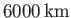. Consequently, it is a good approximation to treat the atmosphere as a relatively thin layer, covering the surface of the Earth, in which the pressure and density are only functions of altitude above ground level,, and the gravitational potential energy per unit mass takes the form, whereis the acceleration due to gravity at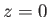. It follows from Equation (13.1) that(13.5)

Now, in an isothermal atmosphere, in which the temperature,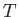, is assumed not to vary with height, the ideal gas equation of state (1.84) yields [cf., Equation (13.3)](13.6)

The previous two equations can be combined to give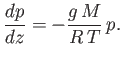(13.7)

Hence, we obtain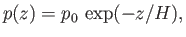(13.8)

whereis atmospheric pressure at ground level, and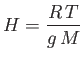(13.9)

is known as the isothermal scale height of the atmosphere. Using the values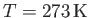(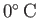),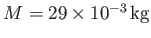, and, which are typical of the Earth's atmosphere (at ground level), as well as, we find that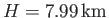. Equations (13.6) and (13.8) yield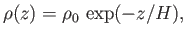(13.10)

whereis the atmospheric mass density at. According to Equations (13.8) and (13.10), in an isothermal atmosphere, the pressure and density both decrease exponentially with increasing altitude, falling toof their values at ground level when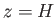, and to only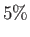of these values when.Next: Adiabatic Atmosphere Up: Equilibrium of Compressible Fluids Previous: Introduction
Richard Fitzpatrick 2016-03-31